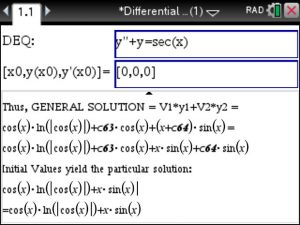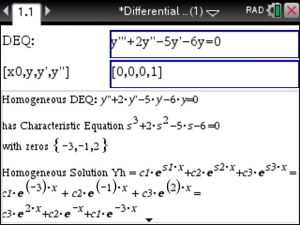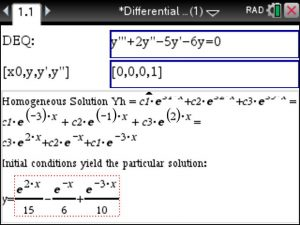# ▷Differential Equation Solver for the TiNspire CAS – Step by Step

The Differential Equation Solver using the TiNspire provides Step by Step solutions. Launch the Differential Equations Made Easy app at www.Tinspireapps.com . Here are 2 examples:

1. Solve a 2. order non-homogeneous Differential Equation using the Variation of Parameter method. Just enter the DEQ and optionally the initial conditions as shown below. First, the solution to the homogeneous Diff Eqn is found using the characteristic equation.Next , the general solution is found by means of Variation of Parameters is found, and every step along the way is shown:2. Solve a 3. order homogeneous Differential Equation. Just enter the DEQ and optionally the initial conditions as shown below. Again, the solution to the homogeneous Diff Eqn. is found using its characteristic equation.Lastly, the Initial Conditions are used to find the Particular Solution. See below.Posted on Categories differential equation, tinspirecx